Nephropathology

 Case 81 With discussion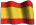Versión en Español

CASE 81 (November 2012)

Clinical information

The patient is a 41-year-old man with a incidental tumoral mass in the upper pole of the right kidney. This mass measured 4.2 x 4.0 x 4.0 cm. The mass was resected by partial nephrectomy.

We received the case in consultation and we do not have gross images.

See the microscopic images.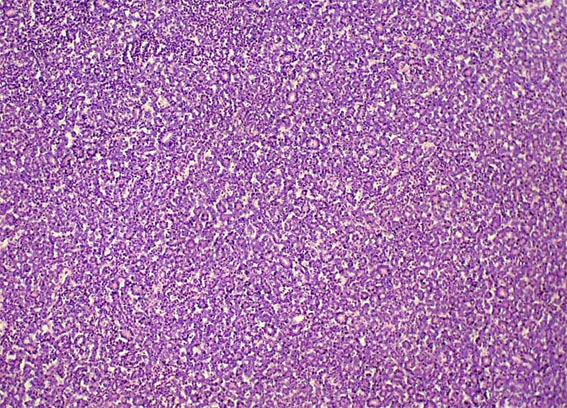Figure 1. H&E, X100.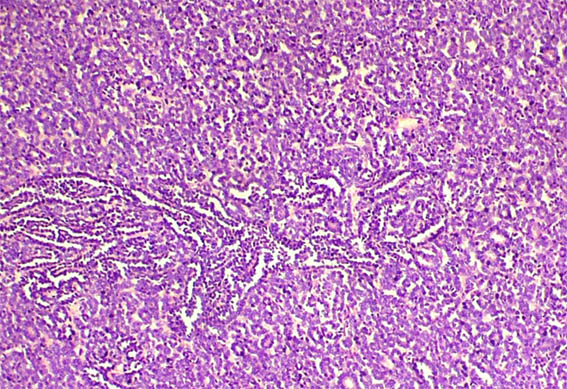Figure 2. H&E, X100.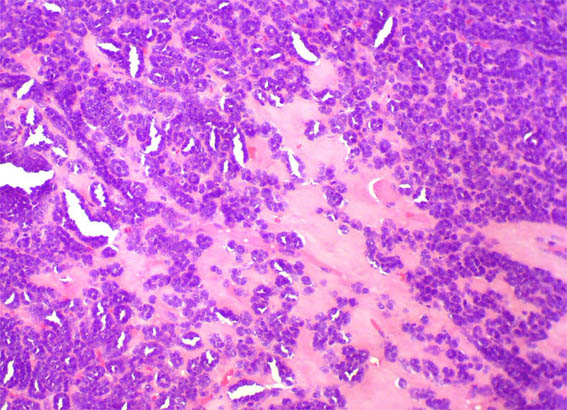Figure 3. H&E, X200.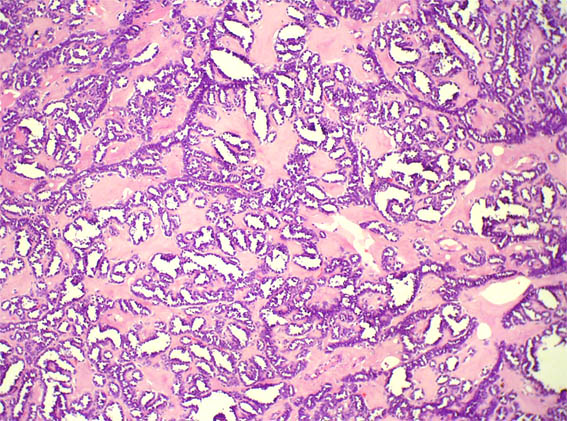Figure 4. H&E, X100.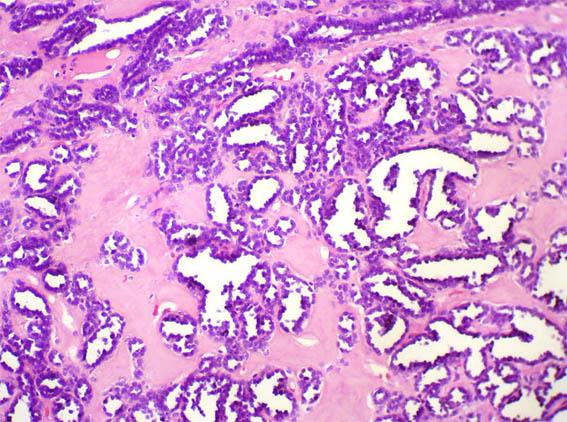Figure 5. H&E, X200.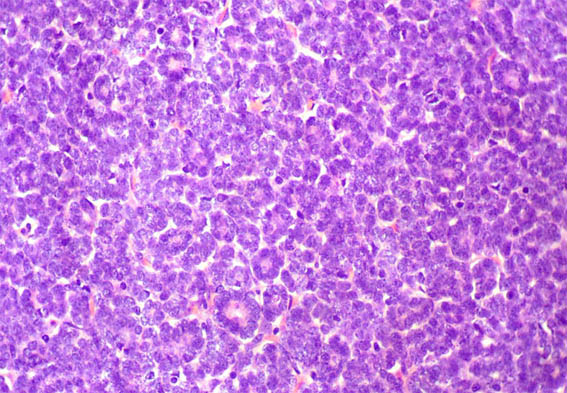Figure 6. H&E, X400.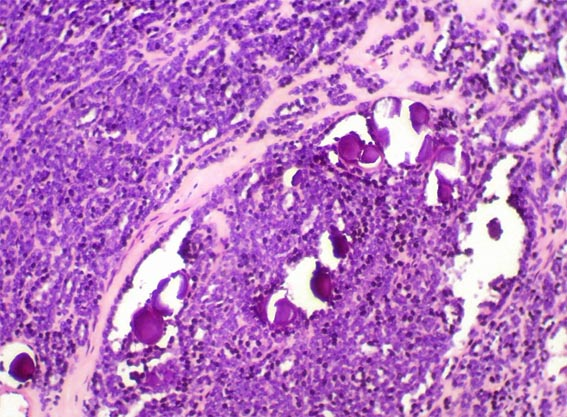Figure 7. H&E, X200.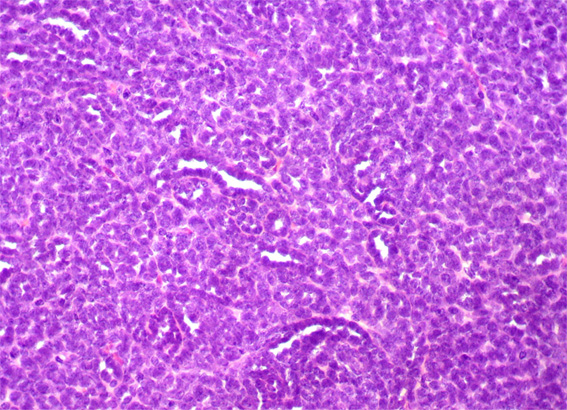Figure 8. H&E, X200.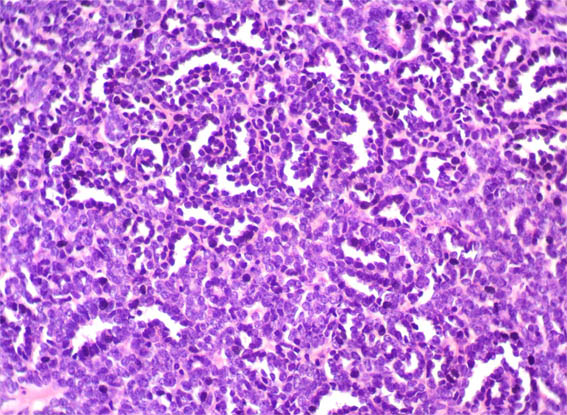Figure 9. H&E, X200.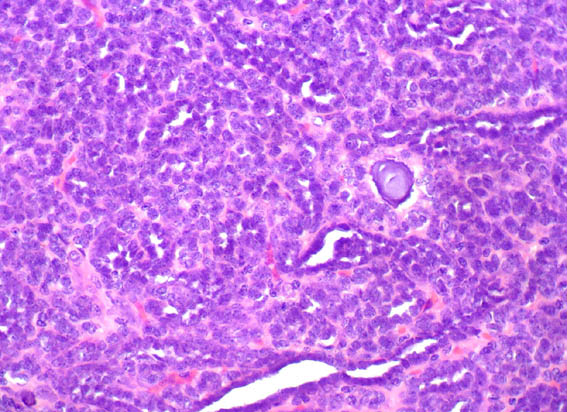Figure 10. H&E, X400.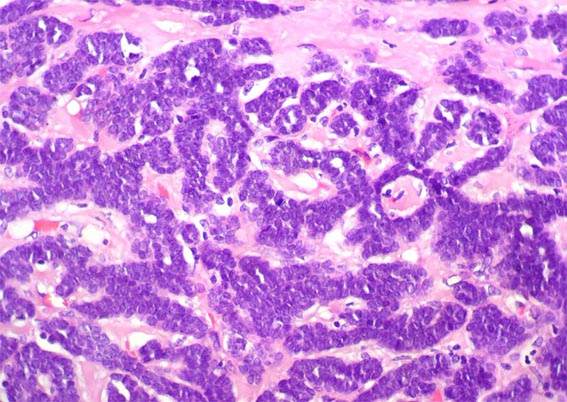Figure 11. H&E, X400.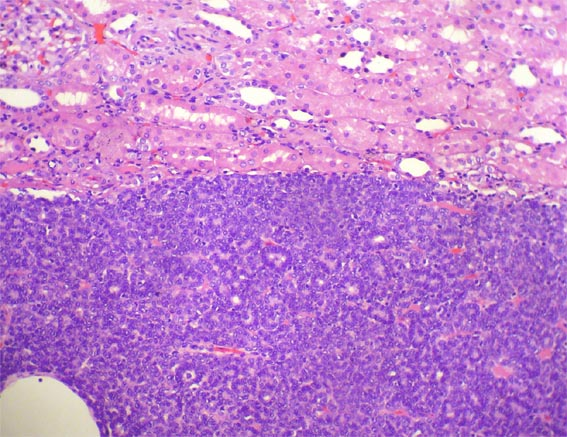Figure 12.H&E, X200.